Smartick is an advanced online program that teaches kids math and coding in only 15 min. a day

Jan02

# How to Multiply by 2 and 3 Digit Numbers

In this post, we are going to learn how to multiply by two-digit and three-digit numbers.

Before we begin, let’s review what the multiplication terms are:

• Factors: Factors are numbers that are being multiplied.
• Product: The product is the result of the multiplication.
• Multiplicand: The multiplicand is the number (factor) that gets multiplied.
• Multiplier: The multiplier is the number (factor) that you are multiplying by.

Usually, the factor with more digits is written first. Usually, the multiplicand is bigger than the multiplier.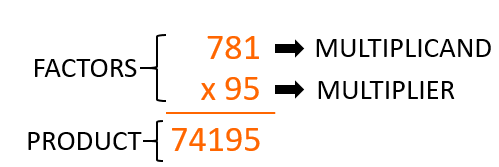Now we are going to see what the steps are in order to multiply by two-digit and three-digit numbers.

First step:  Multiply the ones digit of the bottom factor (multiplier) by the top factor (multiplicand) and write the result on the line below.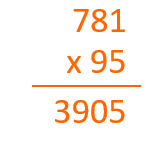Let’s look at an example. If we multiply 781 x 95, the first thing to do is to multiply by 5, which is in the ones place of 95, by each one of the digits of the top factor from right to left, and place the result, 3905, on the line below, as shown in the image.

Second step: Multiply the digit in the tens place of the bottom factor by the top factor and write the result on the line below, but place a 0 in the ones place, since this part of the multiplication is a number of tens.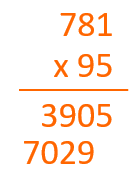We continue with the example. Now we multiply the 9, given that it is in the tens’ place of the bottom factor 95, by the top factor 781. The result, 7029, will have to be written under 3905 but moved one place to the left.

Third step:  Add the products.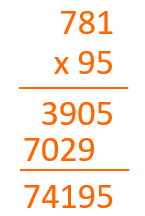As we see in the image we add the products and the result of the multiplication is 74,195.

If the bottom factor (multiplier) is a three-digit number, the result of the multiplication of the hundreds place will be followed by two 0s. Let’s look at another example.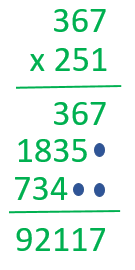If we multiply 367 x 251, the first thing to do is to multiply the digit in the ones place of 251, which is 1, by 367. The result would be 367 and we put it on the line below.

After we multiply the digit in the tens place of 251, which is 5, by 367. The result would be 1835 and we put it on the line below followed by a 0 in the ones place.

Next we multiply the digit in the hundreds place of 251, that is to say, 2, by 367. The result would be 734 and we put it on the line below followed by a 0 in the tens place and a 0 in the ones place.

Finally, we do the addition, and the product is 92,117.

If you want to practice multiplying by 2 and 3 digit numbers and more elementary math, try Smartick for free!

• Jenthsy AlessandraMay 04 2020, 1:40 AM

This helped me a lot with my studies.

• FayyadDec 07 2018, 7:34 AM

This helped me with my homework

• AnyaMar 05 2020, 9:28 PM

Same here

• AnyaMar 05 2020, 9:30 PM

Helped me a lot with my homework

• cali maxamed AadamMar 27 2018, 4:03 AM

good